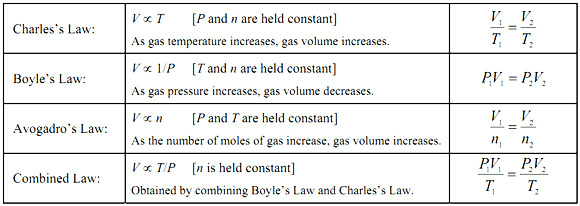# What does the ideal gas law allow a scientist to calculate that the other gas laws do not?

May 26, 2015

There are four gas laws.

Volume is inversely proportional to pressure, or $P V = k$.

Charles' Law

Volume is directly proportional to temperature, or $\frac{V}{T} = k$

Gay-Lussac's or Amonton's Law

Pressure is directly proportional to temperature, or $\frac{P}{T} = k$

Volume is directly proportional to the number of moles, or $\frac{V}{n} = k$

Put them all together, and you get

The Ideal Gas Law

$\frac{P V}{n T} = k$ or $P V = n k T$

We usually replace the constant $k$ with the universal gas constant $R$ and write the equation as

$P V = n R T$The Ideal Gas Law is simply a combination of the other four gas laws.